Intermediate value property not implies continuous

This article gives the statement and possibly, proof, of a non-implication relation between two function properties. That is, it states that every function satisfying the first function property (i.e., intermediate value property) need not satisfy the second function property (i.e., continuous function)
View a complete list of function property non-implications | View a complete list of function property implications
Get more facts about intermediate value property|Get more facts about continuous function
EXPLORE EXAMPLES YOURSELF: View examples of subgroups satisfying property intermediate value property but not continuous function|View examples of subgroups satisfying property intermediate value property and continuous function

Definition

It is possible to have a real-valued function defined on all real numbers that satisfies the intermediate value property but that is not a continuous function, i.e., there is at least one point where it is not continuous.

Proof

Example of the sine of reciprocal function (isolated discontinuity)

For further information, refer: sine of reciprocal function

Consider the function that equals the sine of reciprocal function for nonzero values and is zero at zero. Explicitly:$f(x) := \left\lbrace \begin{array}{rl} \sin(1/x), & x \ne 0 \\ 0, & x = 0 \end{array}\right.$

NOTE: For the value at zero, we could choose any value in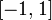$[-1,1]$ instead of 0 and all the arguments below would work.

Here is the graph, at various levels of magnification:

No limit at zero, hence discontinuous at zero (continuous elsewhere)

The function takes the value 1 at all points of the form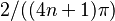$2/((4n + 1)\pi)$ for integers$n$, and takes the value -1 at all points of the form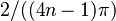$2/((4n - 1)\pi)$ for integers$n$. We can use this to see that the function takes the values 1 and -1 for points arbitrarily close to 0. It therefore cannot have a limit at 0. (In the$\epsilon-\delta$ definition, picking any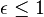$\epsilon \le 1$ will yield trouble because no matter how small the$\delta$ we pick, the value of the function cannot be trapped in an interval of size$\epsilon$ because it must visit both 1 and -1).

Intermediate value property held everywhere

As noted above, the function takes values of 1 and -1 arbitrarily close to 0. It is also continuous on the right of 0 and on the left of 0. It therefore satisfies the intermediate value property on either side of 0, and in particular, takes all values in the interval$[-1,1]$ arbitrarily close to zero on either side. Also, the range of the function is$[-1,1]$, so basically the function takes its full range on inputs arbitrarily close to zero. Based on this, we see that the function satisfies the intermediate value property between 0 and any point, and also across zero (between a positive and negative value). Since it already satisfies the intermediate value property on either side of zero, it satisfies the intermediate value property globally.

Thinking to motivate the example

Our normal toy examples of discontinuous functions use jump discontinuities, with a function jumping from one value to another. Jump discontinuities, however, will also fail the intermediate value property.

To come up with an example that satisfies the intermediate value property at and around a point, we need the function value near the point to cover the entire neighborhood of the function value at a point -- and to keep doing so as we zoom in closer and closer to the point. However, for it to not be continuous at the point, we also don't want the function value to be trapped in small neighborhoods. In other words, we want the function value to keep oscillating by some constant or increasing amount as we zoom in closer and closer to our point of interest.

We already have the example of a sine function that is oscillating, but its oscillations have a constant period. We want to take this same oscillation behavior and compress it to happen increasingly frequently near a point. Using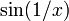$\sin(1/x)$ near zero accomplishes this.